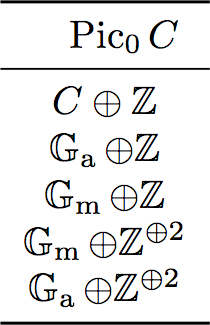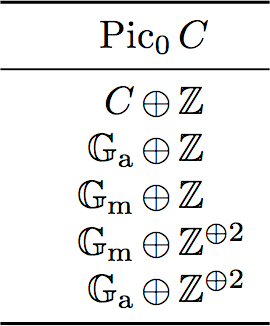This is a little LaTeX hack that I would like to document. For something that I am writing right now I have a big table, where one column contains a certain abelian group. All these groups are direct sums of two other abelian groups. Wouldn't it be nice if the column was aligned on the $\oplus$? At the end of this post there is a comparison to see what I mean in practice.

These abelian groups are the Picard group of certain singular curves, and they are direct sums of $\mathrm{Pic}_0$ and a number of copies of $\mathbb{Z}$ depending on the number of irreducible components. But this is not very important for the remainder of the post.

Using the dcolumn package it is possible to align columns on a decimal point. Luckily, this decimal point can be chosen in a rather broad sense. The column specifier

tells you that we wish to align on the + symbol in the LaTeX code, and that we wish to display \oplus as our decimal point. The -1 is not important for us, because we don't have actual decimals.

A few things to keep in mind:

• the dcolumn package automatically turns everything into math mode, hence you don't need to use 's in this particular column; • so if you need to align actual text using this method, you can always wrap things in \text{}; • if you have a column header, you do not with to apply the dcolumn column specifier to it, which is possible by using \multicol{1}{c}{...}. Compare:\begin{tabular}{c} \toprule \multicolumn{1}{c}{\quad\Pic_0C$} \\ \midrule$C\oplus\mathbb{Z}$\\$\Ga\oplus\mathbb{Z}$\\$\Gm\oplus\mathbb{Z}$\\$\Gm\oplus\mathbb{Z}^{\oplus2}$\\$\Ga\oplus\mathbb{Z}^{\oplus2}$\\ \bottomrule \end{tabular}\begin{tabular}{D{+}{\,\oplus\,}{-1}} \toprule \multicolumn{1}{c}{$\quad\Pic_0C\$} \\ \midrule C+\mathbb{Z} \\ \Ga+\mathbb{Z} \\ \Gm+\mathbb{Z} \\ \Gm+\mathbb{Z}^{\oplus2} \\ \Ga+\mathbb{Z}^{\oplus2} \\ \bottomrule \end{tabular}

Notice that I had to move the column header to the right a little. I should figure out a better solution, especially because this creates a little extra margin on the left. But this nitpickery has gone on long enough now.